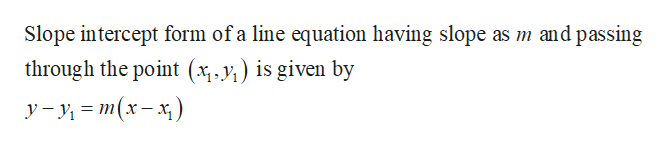# Use slope intercept form to write the equation of the line with the given properties. Write each equation in slop intercept form1.)  m=0, passing through P(2,-4)

Question

Use slope intercept form to write the equation of the line with the given properties. Write each equation in slop intercept form

1.)  m=0, passing through P(2,-4)

check_circleExpert Solution
Step 1

Formula:...help_outlineImage TranscriptioncloseSlope intercept form of a line equation having slope as m and passing through the point (x,y) is given by У -у- т(x - к) fullscreen

### Want to see the full answer?

See Solution

#### Want to see this answer and more?

Solutions are written by subject experts who are available 24/7. Questions are typically answered within 1 hour*

See Solution
*Response times may vary by subject and question
Tagged in

### Other